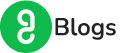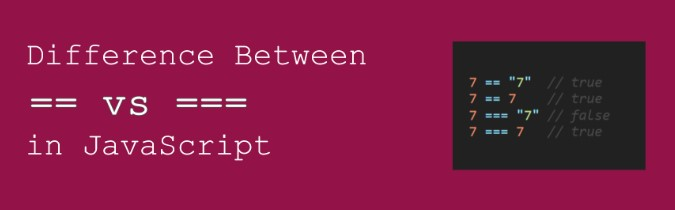# 4 Key Differences Between == and === Operators in JavaScriptJavaScript, which is the most demanding and high-paying programming language comes with various benefits. Not only build web applications, but JavaScript also helps in developing game applications, web servers, etc. There are various elements in JavaScript that can be used during the implementation of projects. One of them is – Operators in JavaScript.

Operators in JavaScript play an important role to manipulate data. To perform operations in data, we use operators which modify it and can be used in programs. From adding two numbers to comparing two values, every single operation can be performed using operators in JavaScript.

In this blog, we’ll be focusing majorly on comparison operators which include == and ===. This topic is the most confusing one as developers get confused as to which one to choose and on which condition. So, let’s discuss the differences between the == and === operators in JavaScript.

## Operators in JavaScript

While using JavaScript, you can work on operators which help you to perform operations such as calculations, assigning values to operands, comparing values, working on logical functions, etc. Operators in JavaScript let you perform such operations in a few seconds while coding. Some of the operators in JavaScript are –

• Assignment Operators
• Arithmetic Operators
• Comparison Operators
• Logical Operators
• Bitwise Operators

We’ll be focusing much on comparison operators as this blog is majorly focused on == and === operators which fall under the category of comparison operators.

### Comparison Operators

Comparison operators let you compare their operands and return a logical value based on the given statement. They are used in logical statements to determine the equality between variables or values. The output for comparison comes in Boolean value i.e., true or false. They are used in decision-making and loops. Also, the operands used in operations can be of data types like numerical, string, logical, or object values.

Some of the symbols which describe the operations are –

## == and === Operators in JavaScript

We’ll be discussing the differences between == and === operators in JavaScript, why should you use and their use cases. Let’s dive deep into them.

### == Operator in JavaScript

== Operator in JavaScript is the abstract equality comparison operator also known as loose equality. It checks whether both the variables being compared are having the same value or not. If both have the same value, irrespective of the data type of the variable (operand), it returns true, else if both have different values, the output comes as false.

Also, here boolean, numbers, and strings are compared by value, not by reference, and if they have the same equivalent value then are considered true. == operator converts the value of the variable to the same type before comparing. This is why it is also called type coercion.

#### Example of == Operator

1. `console.log(42 == 42);`
2. `console.log(21 == '21');`
3. `console.log(21 == '32');`
4. `console.log(true== 0);`

The output of the above-mentioned code:

1. true
2. true
3. false
4. false

Explanations of the output –

Since, the == operator only looks after the values whether they’re the same or not, not the data type of both variables.

In example 1, we have the same values being compared, hence the output is true.
In example 2, we have the same values with different data types, hence the output is true caring only about the variable, not the data type.
In example 3, we have different values being compared, hence the output is false.
In example 4. we have the boolean value true as 0 which is technically not right (true=1, false=0), hence the output is false.

### === Operator in JavaScript

=== Operator in JavaScript is the strict equality comparison operator which only returns true if both the value of the variables (operands) and the data type of the variables are the same, else false. It checks for the value and the data type of the variables and after comparing both sides, if it satisfies both the given conditions, returns true, if not, it returns false.

It strictly compares two values, where it does not perform any type coercion. === operator performs typecasting, it strictly compares two values.

#### Example of === Operators

1. console.log (18===’18’);
2. console.log (isha === ‘isha’);
3. console.log (false === false);
4. console.log (1.9 === 19);

The output of the above-mentioned code:

1. false
2. true
3. true
4. false

Explanations of the output –

Since the === operator looks after both the data and the data type, it checks the data and then the data type of the variables and then produces the output when both conditions get satisfied.

In example 1, only the data is the same, not the data type, hence the output is false
In example 2, we have both the values and data type the same, hence the output is true.
In example 3, we have both the values and data type the same, hence the output is true.
In example 4. we have both the data and the data type different, hence the output is false.

Do check out the JavaScript course which covers all the concepts required for you to have a good grip on it.

## Conclusion

Now that you know the major differences between the == and === operators in JavaScript, you can start implementing these. Where the == operator is used to compare two variables, the === operator compares two variables with the data type. Apart from these operators, we have many other operators as well which can be used in JavaScript coding. Have a deep understanding of the operators in JavaScript and use them precisely while building applications. This can help you develop web applications effectively.

Contact Form

By clicking 'Submit' you Agree to Guvi Terms & Conditions.

Our Trending Courses

Our Learners Work at

## Author BioIsha Sharma
I have two years of experience in the tech industry, one year of experience in Mindtree as a web developer, and 1 year of experience working in GeeksforGeeks as a Technical Content Engineer where I was responsible for writing articles, reviewing other author’s articles, reviewing videos and giving supers for the same.

## Our Live Classes

### Full Stack Development Program (FSD)

Learn Javascript, HTML, CSS, Java, Data Structure, MongoDB & more

### IIT-M Advanced Programming & Data Science Program

Learn Python, Machine Learning, NLP, Tableau, PowerBI & more

### Automation and Testing Program

Learn Selenium, Python, Java, Jenkins, Jmeter, API Testing & more

### UI/UX Program

UX Processes, Design systems, Responsive UI, & more with placement assistance.